# Setting Parameter Limits, Fixing Parameters and Their effects on fitting

You can compare the “fitted” parameters (e.g., playing with different fit options) with the “true” ones (that were used to simulate the histogram, but note that each time you run the simulation, you will get a slightly different histogram).
Of course, for test purposes, you can also use one kind of background for the simulation but then a different type for fitting and so on.

No worries, I’ll play with all. That’s my job.

I noticed the Random filling method , no worries. For instance, all answers below are slightly different than each other.

Can you tell me what MinFCN, NDF, and edm stand for as words here on the terminal results?

I’ll have couple of follow up questions about the discussion for the update. I start to change the code’s approach a bit.

Problems in my mind:

1. TH1F or TH1D, which one is more suitable to the data?

2. I got round of error on gross area 1, how can be fixed? I’ll attach the terminal view.

3. After all is done properly, I may fill the histogram with the integrals from functions instead of the real data. I think it will be pre-defined MODEL based analysis in that way. What is your thought about that?DigitalIntegrationProcedures.cpp (10.4 KB)
(UPDATED)

Co60.txt (3.5 KB)

Cheers.

Try with: `g...->IntegralError(..., 1e-6)`

In your case, I don’t think it makes sense to try to “minimize” the RAM usage by using single-precision, instead of the default double-precision, floating-point format for histograms’ contents.

I forgot. Is this epsilon (1e-6) related to the precision of finding the mean? Should I keep playing with that number until the roundoff error is demolished? I found Moneta mentioned about that.

My conclusion: error disappears as I play with my integral limits. If I go from Lower bin 400 to upper bin 530, I see NO ERROR. Thus, it’s a matter of limits and the ability of fitting procedure within those limits. Am I right?

I see the terminal resuls and the fit result DONOT MATCH. First parameters of each gaussian should be the integral of those peaks due to the usage of Gausn, but the results give different outcomes comparison to parameter outcomes.

One more thing is that the broken line is not broken where I set the value. I want it to break at the bin number 462. Why is that?

If `1e-6` is still too small, try `1e-5`.

The position of the “breakpoint” is a fitted parameter.

Yes, I think it is a good idea to start with a “well-known” histogram.

1 Like

What happens when I fix the break point parameter which I needed. Otherwise, it will be wrong.
I fixed it to the bin number 460, and it looks like OK.

However, the roundoff error 18 is so sensitive to the limits I choose. It only worked from 400 to 460 for the 1st peak and from 460 to 430 bin numbers for the 2nd peak.

Do you think that it’s totally OK to play with numbers until getting NO ERROR?

You can “fix” whatever parameter you want.

BTW. Note that the “area” parameter of a “`gausn`” is always its “full” integral. You integrate the function from some “xmin” to some “xmax”, so such “integral” will always be <= “area”.

When you say full integral, it is the value of this first part below: 1/sigma*squareRoot(2Pi) .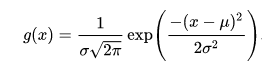The integral, however , is the value below.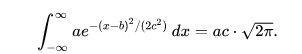A bit confusing. When we fit gausn instead of gaus, do we tell somewhere in the code before it prints out the information, show the area as the 1st parameter. Obviously, the area is NOT equal to normalizating constant.

`gROOT->GetFunction("gaus")->Print();`
`gROOT->GetFunction("gausn")->Print();`

1 Like

It’s nice that I can see quicker like that instead of going through the ROOT website. I wasn’t aware. Thanks for that.

The word constant here is indeed the parameter p0 as we know. The height term in gaus.

That’s the website version.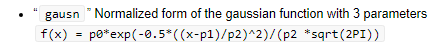To be normalized to 1, p0 here should be set to squareRoot(2xPi )x sigma in gaus.

Gausn is actually different than PDF of the Gaussian function as said on this website.

Ok. let me try my shot to explain my confusion.
In gaus, we give height as parameter, it draws the shape nicely. In gausn, we need to give area to be drawn. By looking at the histogram, I can easily give the hight but not the area. I need to calculate the area from height x sigma x sqrt(2 x Pi) . Isn’t it tiring?

Yeah, I am aware of that.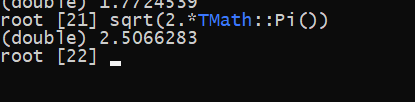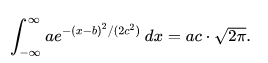Here below, how p0 parameter turns to an area as a fit parameter after fitting?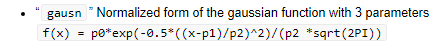When we find the integral and error, we use these two methods:

Should we not use these for gausn? Instead, should we just retrieve the parameter and its error, instead?

@Wile_E_Coyote
From terminal results, if I do gross area - background area with a calculator, it doesn’t match the ROOT net area result.

Is that normal!

Try:

``````{
TF1 *f = (TF1*)gROOT->GetFunction("gausn");
Double_t area = 100., mean = 0., sigma = 1.;
f->SetParameters(area, mean, sigma);
std::cout << "area = " << area << std::endl;
for (Int_t i = 1; i < 10; i++)
std::cout << i << " : " << area - f->Integral(mean - i * sigma, mean + i * sigma) << std::endl;
}
``````

BTW. Make sure that you use:
`total = g1 + g2 + bg`
`bg_g1 = total - g1 = g2 + bg` (i.e., it’s always `>= bg`)
`bg_g2 = total - g2 = g1 + bg` (i.e., it’s always `>= bg`)

1 Like

``````{

TCanvas *c1 = new TCanvas("c1", "c1", 800,400);
c1->Divide(2,1);
c1->cd(1);

TF1 *f = (TF1*)gROOT->GetFunction("gausn");
Double_t area1 = 100., mean1 = 0., sigma1 = 1.;
f->SetParameters(area1, mean1, sigma1);
f->Draw();
std::cout << "area 1 = " << area1 << std::endl;
std::cout << "height 1 = " << area1/ (sigma1*sqrt(2.*TMath::Pi())  ) << std::endl;

Int_t i;
cout << "To see the percentage of the marked area in the range of stated sigma in PDF" << endl;
for (i = 1; i < 10; i++)
std::cout << i << " area 1: "<< area1 << " integral: " <<  f->Integral(mean1 - i * sigma1, mean1 + i * sigma1) << "\t\tThe rest: "  << area1- f->Integral(mean1 - i * sigma1, mean1 + i * sigma1)  << std::endl;

c1->cd(2);

TF1 *f2 = (TF1*)gROOT->GetFunction("gaus");
Double_t height2 = 100., mean2 = 0., sigma2 = 1.;
f2->SetParameters(height2, mean2, sigma2);
f2->Draw();
std::cout << "area 2 = " << sqrt(2.*TMath::Pi()) *height*sigma2 << std::endl;
for ( i = 1; i < 10; i++)
std::cout << i << " : " << f2->Integral(mean2 - i * sigma2, mean2 + i * sigma2) << std::endl;
}
``````

BTW, I am suprised about the gaus plot above. Its x axis range is between + sigma and - sigma when we do not state it explicitly. Anyways.

That’s interesting because I wasn’t imagining the ROOT is calculating it like that. Surely, this calculation should be only valid for common (linked) background line, not for the separate bcg line approach. Right! I guess it won’t be valid if the peaks are way apart from each other, then in this case, we need to define 2 bcg functions. I guess ROOT regards the broken line as continous as if there was 1 line.

Question: When we say g1, don’t we mean the net area + its background ?
Answer: Ohh. NO, it’S not because we use the integral of g1 when we get the NET area of g1.

Namely, g1 and g2 are our NET sginals.That’ amazing to see how easily the ROOT finds the net area.

I made it clear below with my worst drawing.

That’ why we limit ourselves within the g1 limits when we try to retrieve the bcg_g1.

For instance: we use lower and upper bin edges as limits.

double bcg_g1 = bcg->Integral(g1_X_LBE, g1_X_UBE) / BinWidth ;

The “toy MC” (by default) sets the “sigma” parameter = `10` for both “`gausn`” peaks. Try to play with “sigma” = `5` and then with “sigma” = `20` (for both peaks, of course).